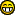News: Follow us on Twitter## The All New FractalForums is now in Public Beta Testing! Visit FractalForums.org and check it out!

 Pages:    Go DownAuthor Topic: Mandelbox with "smooth" conditions  (Read 2608 times) Description: 0 Members and 1 Guest are viewing this topic.
Buddhi
Fractal IambusPosts: 895« on: April 19, 2011, 08:44:49 PM »

I'm not sure whether somebody tried this before, but I converted standard Mandelbox formula into formula without any conditions but with smooth transition functions.

I have replaced condition IF A>B by

where k is a smoothness factor

The code for Mandelbox is following (tested in Mandelbulber):
Code:
double sm = 3.0;

double zk1 = 1.0 / (1.0 + 1.0 / exp(sm * (z.x - par.mandelbox.doubles.foldingLimit)));
double zk2 = 1.0 / (1.0 + 1.0 / exp(sm * (z.x + par.mandelbox.doubles.foldingLimit)));
z.x = z.x * (1.0 - zk1) + (par.mandelbox.doubles.foldingValue - z.x) * zk1;
z.x = z.x * (zk2) + (-par.mandelbox.doubles.foldingValue - z.x) * (1.0 - zk2);

double zk3 = 1.0 / (1.0 + 1.0 / exp(sm * (z.y - par.mandelbox.doubles.foldingLimit)));
double zk4 = 1.0 / (1.0 + 1.0 / exp(sm * (z.y + par.mandelbox.doubles.foldingLimit)));
z.y = z.y * (1.0 - zk3) + (par.mandelbox.doubles.foldingValue - z.y) * zk3;
z.y = z.y * (zk4) + (-par.mandelbox.doubles.foldingValue - z.y) * (1.0 - zk4);

double zk5 = 1.0 / (1.0 + 1.0 / exp(sm * (z.z - par.mandelbox.doubles.foldingLimit)));
double zk6 = 1.0 / (1.0 + 1.0 / exp(sm * (z.z + par.mandelbox.doubles.foldingLimit)));
z.z = z.z * (1.0 - zk5) + (par.mandelbox.doubles.foldingValue - z.z) * zk5;
z.z = z.z * (zk6) + (-par.mandelbox.doubles.foldingValue - z.z) * (1.0 - zk6);

r = z.Length();
double r2 = r * r;

double m_factor1 = fR2 / mR2;
double m_factor2 = fR2 / r2;
double rk1 = 1.0 - 1.0 / (1.0 + 1.0 / exp(sm * (r2 - mR2)));
double rk2 = 1.0 - 1.0 / (1.0 + 1.0 / exp(sm * (r2 - fR2)));
double rk21 = (1.0 - rk1) * rk2;

z = z * (1.0 - rk1) + z * (m_factor1 * rk1);
z = z * (1.0 - rk21) + z * (m_factor2 * rk21);

z = z * scale + constant;
r = z.Length();

As you see there is no any "IF" command.

Bellow there is an example:http://www.fractalforums.com/index.php?action=gallery;sa=view;id=6817

The shape is almost the same, but a little more rounded somewhere

This is other example:http://www.fractalforums.com/index.php?action=gallery;sa=view;id=6792

Of course rendering is much slower but shapes are very interesting somewhereLogged

marius
Fractal LoverPosts: 206« Reply #1 on: April 19, 2011, 09:08:47 PM »

I'm not sure whether somebody tried this before, but I converted standard Mandelbox formula into formula without any conditions but with smooth transition functions.

I have replaced condition IF A>B by
<Quoted Image Removed>

where k is a smoothness factor

Interesting. More organic, H.R. Giger-style appearance.
Nice glow rendering!Logged
msltoe
IteratorPosts: 187« Reply #2 on: April 20, 2011, 04:03:57 AM »

Buddhi,

I've experimented with smooth transitions for my favorite YZ symmetrized Mandelbulbs, but found in those cases I had to use sharp functions to prevent artifacts.
I've looked at and others have mentioned the use of sin(x) for reflections  but found that the sine function is too smooth.
BTW, the formula in your post has a typo : it should read: 1/1+exp... but I see you coded it up correctly.

If you want you can post the smooth and non-smooth side by side so we can see the difference. I've been concerned though, that any smooth transition actually causes conformality violations. In other words, the if-test formula, even though it's discontinous in first derivative, preserves angles as you zoom in.

-mikeLogged
Buddhi
Fractal IambusPosts: 895« Reply #3 on: April 20, 2011, 07:15:46 AM »

Buddhi,

I've experimented with smooth transitions for my favorite YZ symmetrized Mandelbulbs, but found in those cases I had to use sharp functions to prevent artifacts.
I've looked at and others have mentioned the use of sin(x) for reflections  but found that the sine function is too smooth.
BTW, the formula in your post has a typo : it should read: 1/1+exp... but I see you coded it up correctly.

If you want you can post the smooth and non-smooth side by side so we can see the difference. I've been concerned though, that any smooth transition actually causes conformality violations. In other words, the if-test formula, even though it's discontinous in first derivative, preserves angles as you zoom in.

-mike

You are right, There was a mistake and I have also overcomplicated a little this formula. I forgot that exp(x) = 1/exp(-x)It could be:Logged

DarkBeam
Global Moderator
Fractal SeniorPosts: 2512Fragments of the fractal -like the tip of it« Reply #4 on: April 21, 2011, 06:41:47 PM »

If you want you can post the smooth and non-smooth side by side so we can see the difference. I've been concerned though, that any smooth transition actually causes conformality violations.

Too bad it's true - I tried many times to write a smoothed Barnsley using sigmoids like these and they all give weird Julias due to the non conformality.Logged

No sweat, guardian of wisdom!
knighty
Fractal IambusPosts: 819« Reply #5 on: May 22, 2011, 03:02:08 PM »

I'm not sure whether somebody tried this before, but I converted standard Mandelbox formula into formula without any conditions but with smooth transition functions.

I did. But your results are much better.
There is also this one.Logged
 Pages:    Go Down
 Related Topics Subject Started by Replies Views Last post"Smooth" folding 3D Fractal Generation knighty 13 4786September 18, 2011, 12:23:53 PM by DarkBeamSpecial "offset" and "rotate" options from Fractallab possible in M3d-formula? Mandelbulb 3d « 1 2 » SaMMy 18 13876April 05, 2011, 12:20:52 PM by DarkBeamRotated "Mandelbox surface" Amazing Box, Amazing Surf and variations Kali 0 1823July 23, 2011, 06:58:51 PM by KaliA "smooth" transition ... Mandelbulb 3d « 1 2 » DarkBeam 19 3183September 28, 2011, 04:41:27 PM by DarkBeam"Futuristic City Under Construction" Mandelbox Flight Movies Showcase (Rate My Movie) mcsoftware 0 459February 03, 2016, 07:37:18 PM by mcsoftware